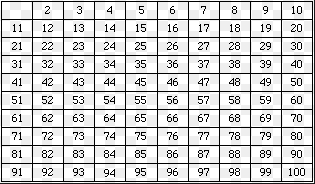Courses

# Introduction to Prime Numbers GMAT Notes | EduRev

## GMAT : Introduction to Prime Numbers GMAT Notes | EduRev

The document Introduction to Prime Numbers GMAT Notes | EduRev is a part of the GMAT Course Quantitative Aptitude for GMAT.
All you need of GMAT at this link: GMAT

What is a Prime Number?
A prime number is a positive integer having exactly two factors. If p is a prime, then it’s only factors are necessarily 1 and p itself. Any number which does not follow this is termed as composite numbers which means that they can be factored into other whole numbers.

• First Ten Prime Numbers:

The first ten primes are 2, 3, 5, 7, 11, 13, 17, 19, 23, 29.

Note: It should be noted that 1 is a non-prime number.

Here, all the concepts of prime numbers are covered along with various examples. The key concepts that are covered here include:

• Prime numbers 1-100
• Why 1 is Not a Prime Number?
• Smallest Prime Number
• Largest Prime Number
• How to Find Prime Numbers Easily?
• Properties of Prime Numbers

Prime Numbers 1 to 100 List

The table below shows the list of prime numbers from 1 to 100.Why 1 is not a prime number?
Conferring to the definition of the prime number which states that a number should have exactly two factors for it to be considered as a prime number. But, number 1 has one and only one factor which is 1 itself. Thus, 1 is not considered as a Prime number.

Smallest Prime Number
The smallest prime number as defined by modern mathematicians is 2. To be prime, a number must be divisible only by 1 and the number itself which is fulfilled by the number 2.

Largest Prime Number
As of January 2016, the largest known prime number is 2{77,232,917} – 1, a number with 23,249,425 digits. It was found by the Great Internet Mersenne Prime Search (GIMPS).

How to Find Prime Numbers?
Let us choose any random number “A.” We can find whether this number is a prime number or not using the following method-

Find the number k, where k2>A
If A is divisible by any prime number less than k, then A is a non-prime number, otherwise, A is a Prime number. Know how to find prime numbers online from 1 to 100.

Example Questions:
Example 1: Is 10 a Prime Number?
Answer – No, because it can be divided evenly by 2 or 5, 2×5=10, as well as by 1 and 10.
Example 2: Is 19 a Prime Number?
Answer – First we need to find the number k, such that k2>19.

Thus the value of k comes out to be 5, as 52 = 25.

The prime numbers less than 5 are 2 and 3.

Clearly, we can see that 19 is not divisible by both 2 and 3.

Therefore, 19 is a prime number.

Note- It should be noted that an integer suppose, P > 1 is termed as a prime number when its only divisors are 1 and P.
Any numeric m > 1 which is not a prime is termed as a composite.

Each composite number can be factored into prime factors, and individually all of these are unique in nature.

Properties of Prime Numbers
Some of the properties of primes are:

• Every n > 1 is can be divided by some prime.
• The product is divisible by a prime ‘p’ only when ‘p’ divides one of the factors.
• A prime ‘p’ is one or the other relatively prime to a number ‘n’ or divide it.

Each composite number can be factored into prime factors, and individually all of these are unique in nature.

Offer running on EduRev: Apply code STAYHOME200 to get INR 200 off on our premium plan EduRev Infinity!

## Quantitative Aptitude for GMAT

85 videos|99 docs|176 tests

,

,

,

,

,

,

,

,

,

,

,

,

,

,

,

,

,

,

,

,

,

;